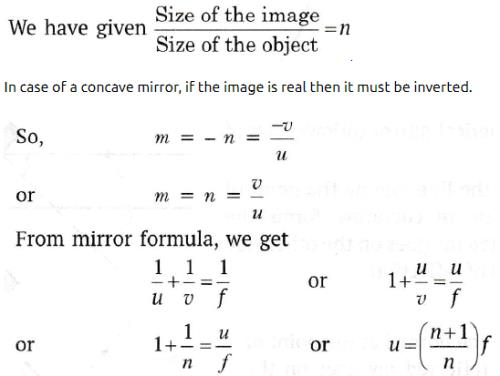Courses

# Test: Mirror Formulae

## 15 Questions MCQ Test General Science(Prelims) by IRS Divey Sethi | Test: Mirror Formulae

Description
This mock test of Test: Mirror Formulae for UPSC helps you for every UPSC entrance exam. This contains 15 Multiple Choice Questions for UPSC Test: Mirror Formulae (mcq) to study with solutions a complete question bank. The solved questions answers in this Test: Mirror Formulae quiz give you a good mix of easy questions and tough questions. UPSC students definitely take this Test: Mirror Formulae exercise for a better result in the exam. You can find other Test: Mirror Formulae extra questions, long questions & short questions for UPSC on EduRev as well by searching above.
QUESTION: 1

### When a mirror is rotated through an angle θ, the reflected ray from it turns through an angle of

Solution:

Consider a plane-mirror and a fixed incident ray of light reflecting from the surface at an angle θi. Before the mirror has rotated, the angle of incidence is θ as is the angle of reflection. If the mirror is rotated through an angle φ the normal is rotated by an angle &phi and thus the angle of incidence increases to θ+φ. Therefore, the angle of reflection must also increase by φ to θ+φ The difference between the final angle of reflection and the initial angle of reflection and is 2φ.
Hence, For a fixed incident ray, the angle of the reflection is twice the angle through which the mirror has rotated: thetaf= 2φ

QUESTION: 2

### An object is placed 60 cm in front of a concave mirror. The real image formed by the mirror is located 30 cm in front of the mirror. What is the object’s magnification ?

Solution:

u = -60 cm, v = -30 cm

m = – v/u = – (-60)/-30 = -0.5

QUESTION: 3

### A concave mirror of focal length ‘f’ produces an image ‘n’ times farther than the object. If the image is real, then the distance of the object from the mirror is:​

Solution:

The correct option is B.QUESTION: 4

If the magnification has a negative sign, the image formed by the concave mirror must be

Solution:

We know that, if the magnification value is negative sign in the concave mirror, then the image will be real and inverted. Especially, when you come to concave mirror, the images are formed at the left of the mirror. So, it forms real and inverted.

QUESTION: 5

If the magnification has a positive sign, the image formed by the concave mirror must be

Solution:

It can either be a convex mirror or concave mirror. It depends upon the position of the object. u is always negative so in order to get magnification positive v must be negative. If the object is kept between pole and the focus of the mirror, then it will form a virtual image behind the mirror and v will be +.

QUESTION: 6

According to the new cartesian sign convention,

Solution: Whether the focal length of a concave mirror is taken as positive or negative depends on the convention being followed. As per the New Cartesian sign convention, The object is placed on the left of the mirror. Distances are measured from the pole of the mirror.
QUESTION: 7

When the image is of double size as the object, the magnification is

Solution:

Magnification is the ratio of height of the image to the height of the object. If the image size is double of object size, then the magnification is equal to two.

QUESTION: 8

If an object is 14 cm in front of convex mirror and image distance is 5.8 cm then it's focal length is

Solution:

1/f = 1/v - 1/u
1/ f = 1/ 5.8 - 1/14
F   = -4. 1 cm

QUESTION: 9

The mirror formula is valid for

Solution:
QUESTION: 10

When the image is of the same size as the object, the magnification is

Solution:
QUESTION: 11

Which one is correct as the new cartesian sign conventions ?

Solution:

Object is always kept on the left side of the mirror therefore its distance is always negative when taken from left of pole.

QUESTION: 12

An object is placed on the principal axis of a spherical mirror. The object distance u is:

Solution:
QUESTION: 13

The unit of linear magnification is

Solution:

Magnification is thr ratio of size of image to size of object and since it is a ratio of tel similar quantities hence it is unitless.

QUESTION: 14

An object 2 cm high is placed at a distance of 15 cm from a concave mirror which produces an inverted image 4 cm high. Find the position of the image.

Solution:

f=-10cm,u=-15cm, 1/v=1/u-1/f
[-15-(-10)]/150=-1/30,so, v=-30 cm. So, the image is formed in the same side of the object at a distance of 30 cm.

QUESTION: 15

The magnification of a convex mirror is always:

Solution:

The image formed by a convex mirror is always smaller in size than the object; hence its magnification is less than 1.